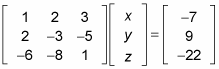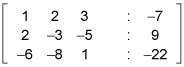##### Pre-Calculus Workbook For DummiesAn alternative to writing a system of equations as the product of a coefficient matrix and variable matrix equaling an answer matrix is what's known as augmented form; this is where the coefficient matrix and the answer matrix are written in the same matrix, separated in each row by colons. This setup makes using elementary row operations to solve a matrix much simpler because you only have one matrix on your plate at a time (as opposed to three!).

Mathematicians are a very lazy bunch, and they like to write as little as possible. Using augmented form cuts down on the amount that you have to write. And when you're attempting to solve a system of equations that requires many steps, you'll be thankful to be writing less! Then you can use elementary row operations to get the solution to your system.

Consider this matrix equation:Written in augmented form, it looks like this: